ENGR338 Lab 2022 Fall
Lab 6
More gates

NAND, NOR , XOR, AND FULL ADDER

Introduction: This lab uses Electric VLSI and LTSpice to simulate pmos and nmos gates in order to create an inverter.

Materials: LTSpice, Electric VLSI

Methods: First, a ring oscilator was created by putting 11 inverters in a loop.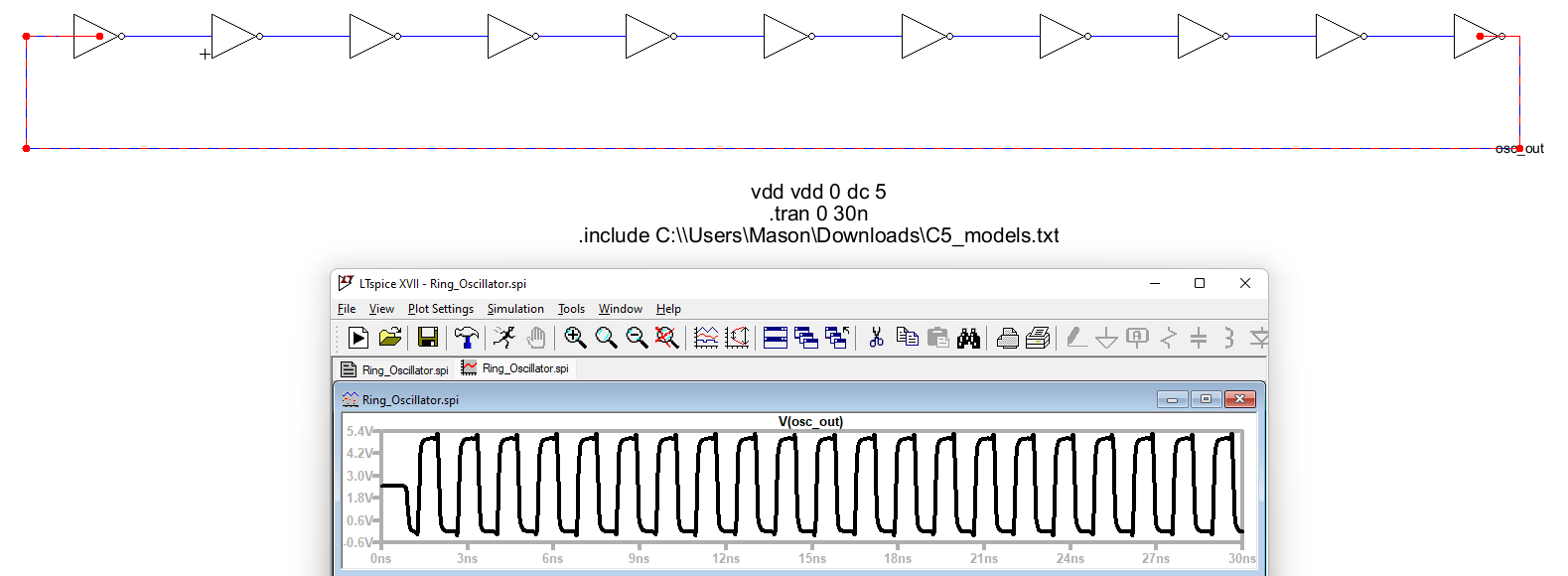Figure 1. Ring oscilator schematic and simulation.

The same circuit was then created using busses as seen in Figure 2.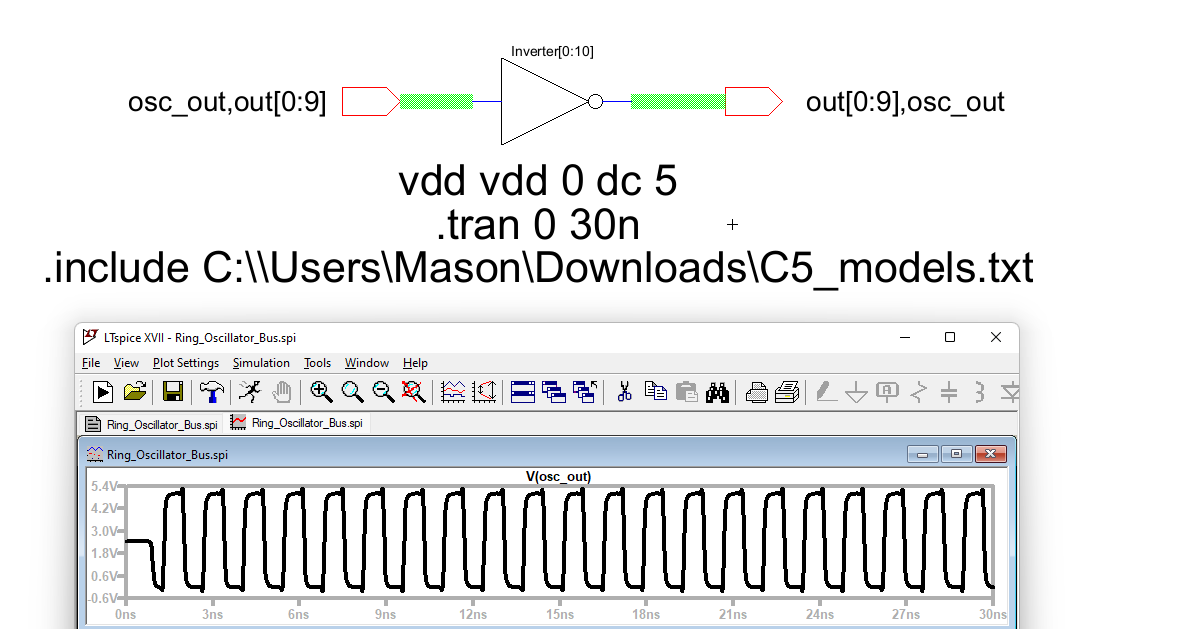Figure 2. Ring oscilator using busses.

The ring oscilator was then laid out and the NCC and DRC matched and passed as seen in Figure 3.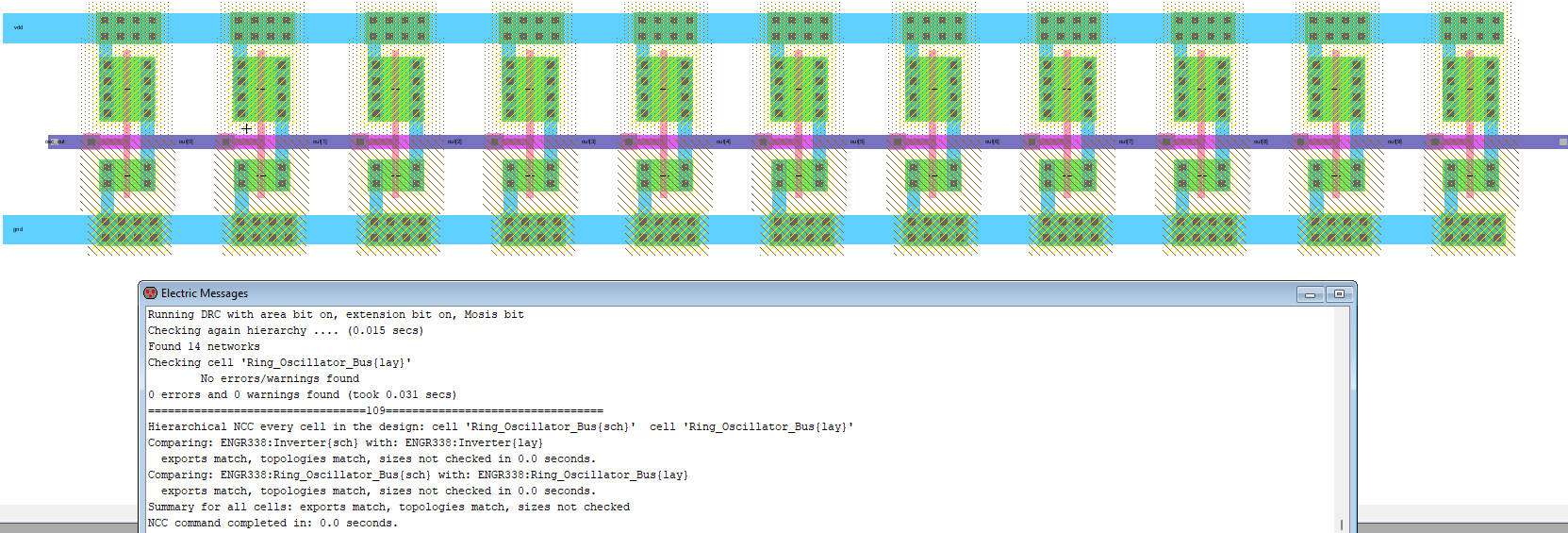Figure 3. Ring oscilator layout.

An 8 bit and gate was created using the same process of busees and can be seen in Figure 4.Figure 4. 8-bit and gate schematic.

The icon was then used to simulate the 8-bit and gate.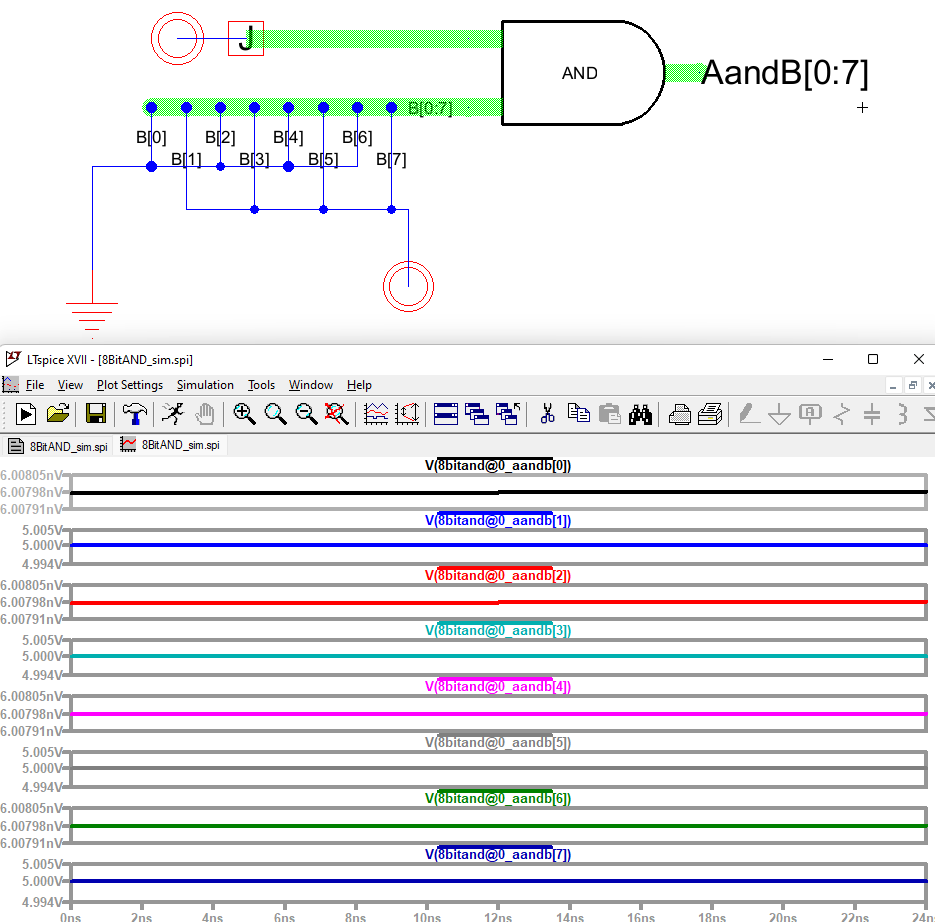Figure 5. 8-bit and gate simulation, even pins are grounded and odd pins are powered.

This process was repeated flipping the top pins from VDD to gnd as seen in Figure 6.Figure 6. Simulated 8-bit and gate with top pin shorted to ground showing the logic is right.

A layout was then created for the 8-bit and gate by copying the and gate layout in an array and then wiring the input and outputs as seen below in Figure 7.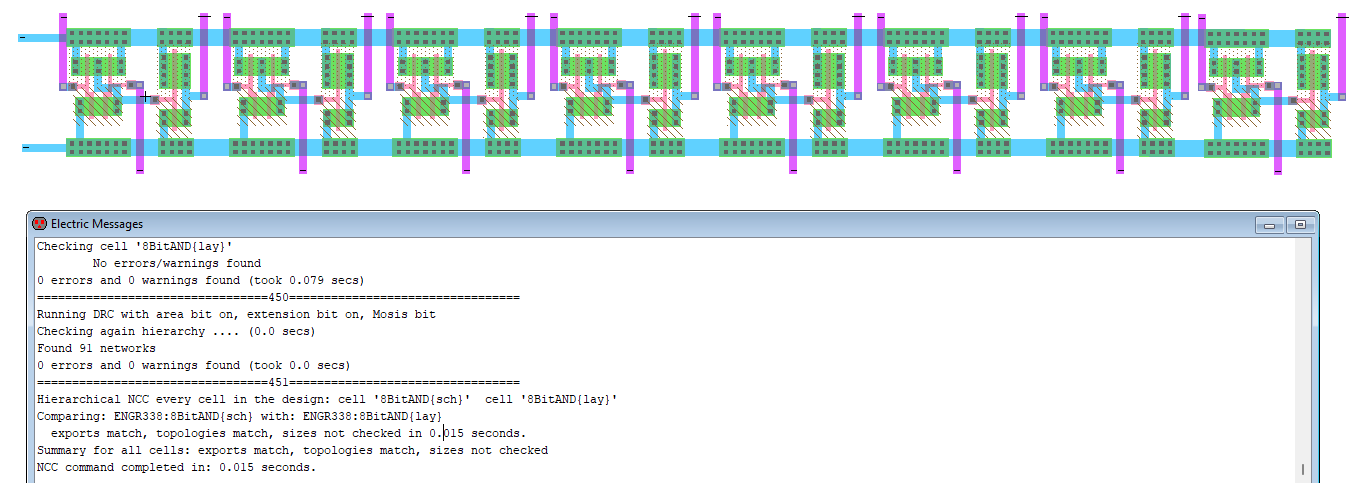Figure 7. 8-bit and gate layout with clean NCC and DRC.

This same process was done for an 8-bit OR gate.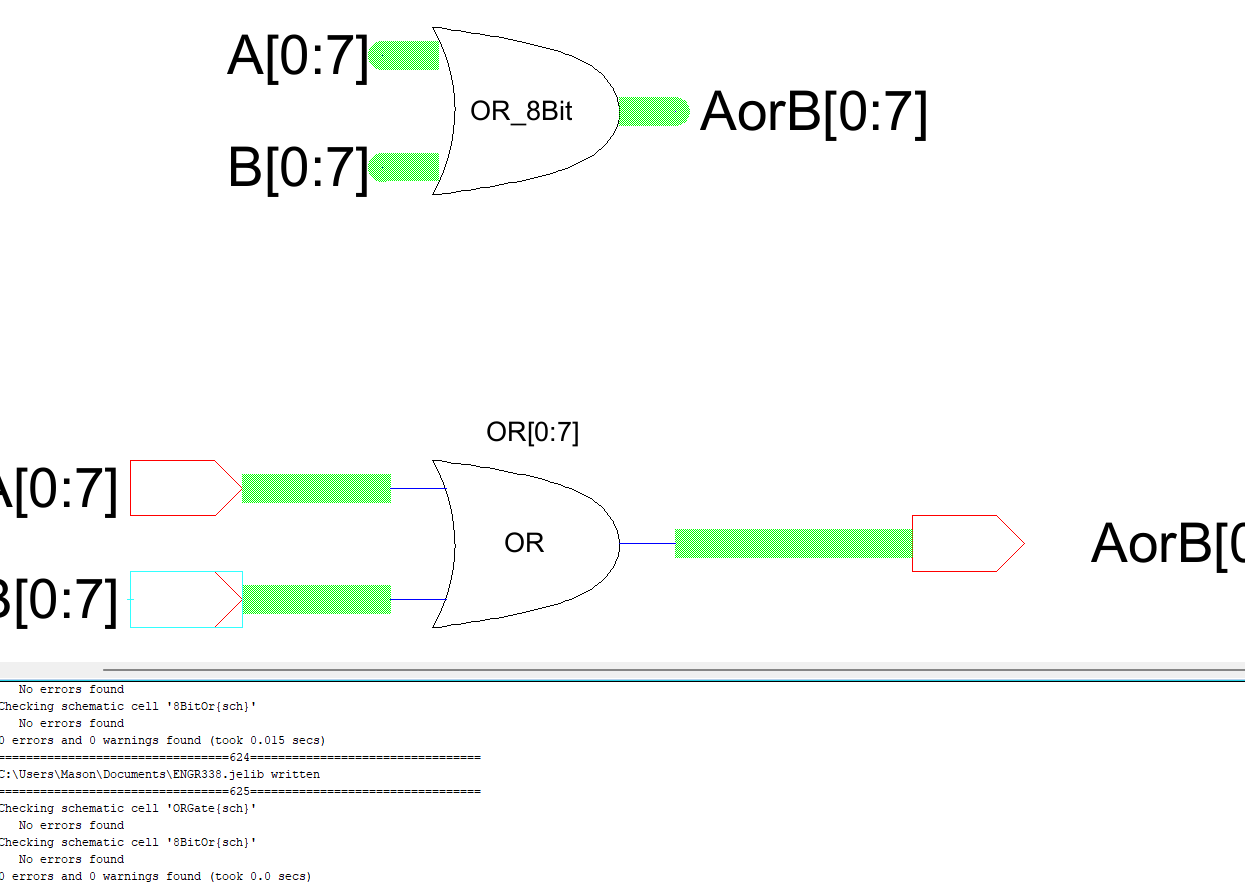Figure 8. 8-bit OR gate using busses with no DRC errors.

The OR gate was then laid out the same way as the AND gate using an array and then routing the inputs and outputs through metal 2.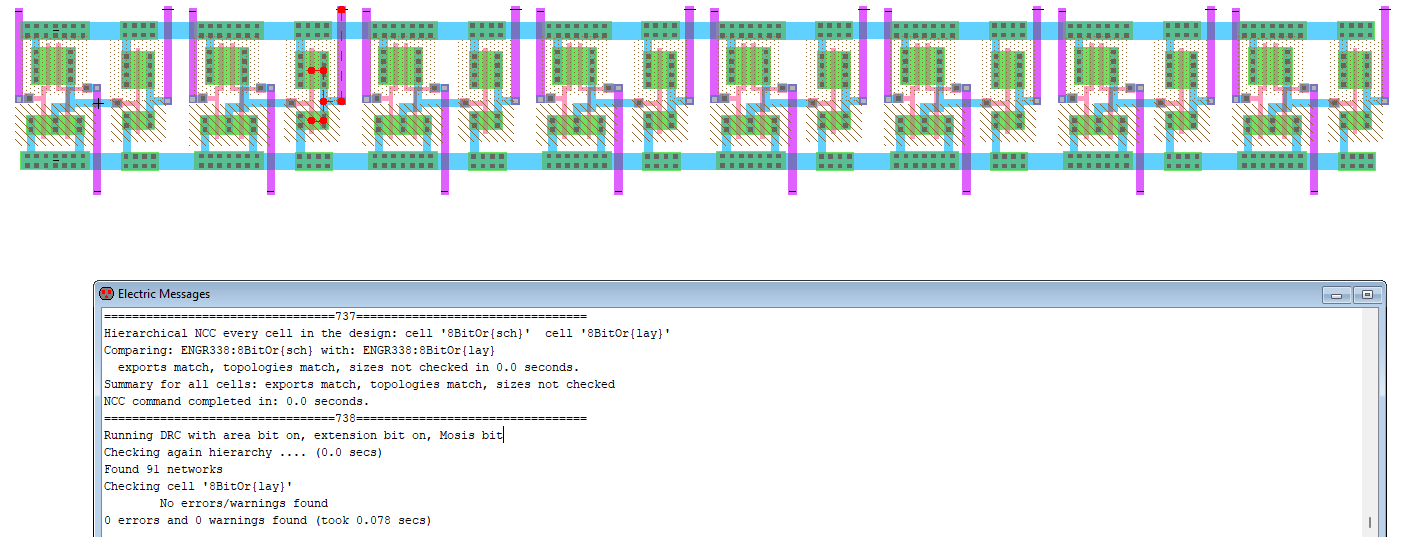Figure 9. 8-bit or gate laiyout with NCC and DRC check complete.

The same process was completed for NAND and NOR gates. The schematics worked the exact same but the layouts were actually easier since they didn't include an extra NMOS and PMOS for the inverters. The schematics can be seen below in Figure 10.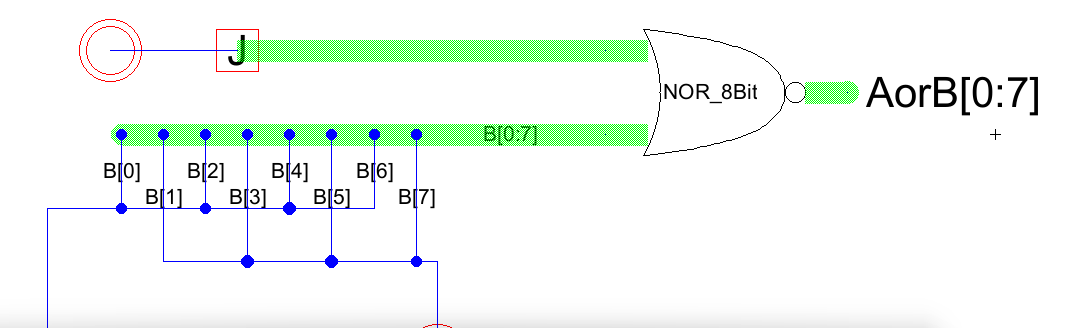Figure 10. 8-bit Nand and Nor gate icons created using busses the same as the And and Or gates above.

These gates were then both simulated(in the results section) and laid out as done earlier with the other gates. This was trivial as it was the same as the And and Or gates just without the inverter from the outputs. The layouts can be seen below in Figure 11.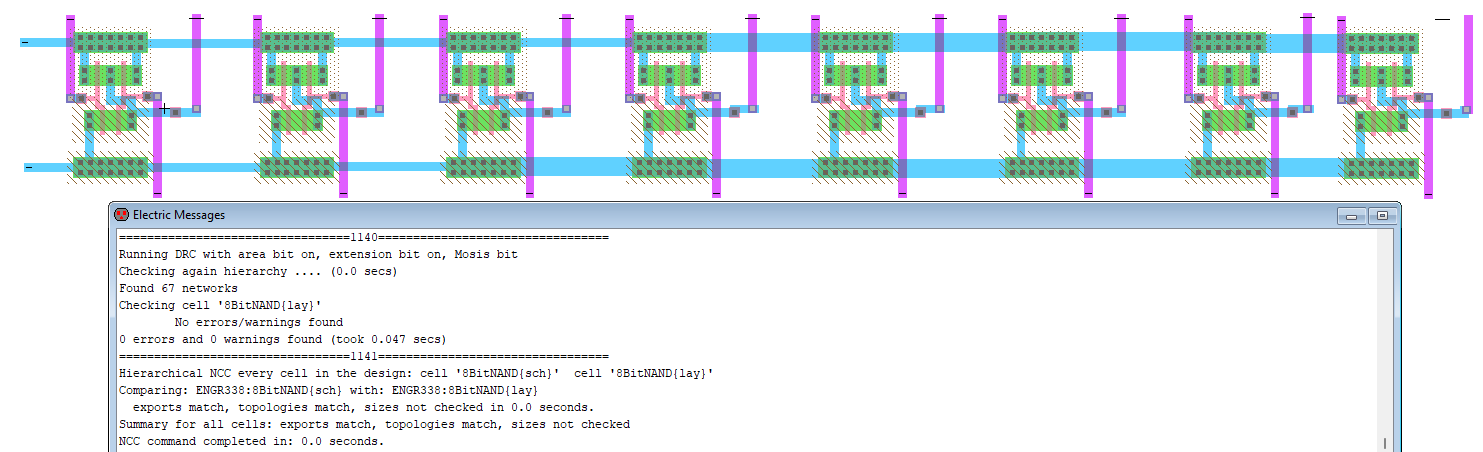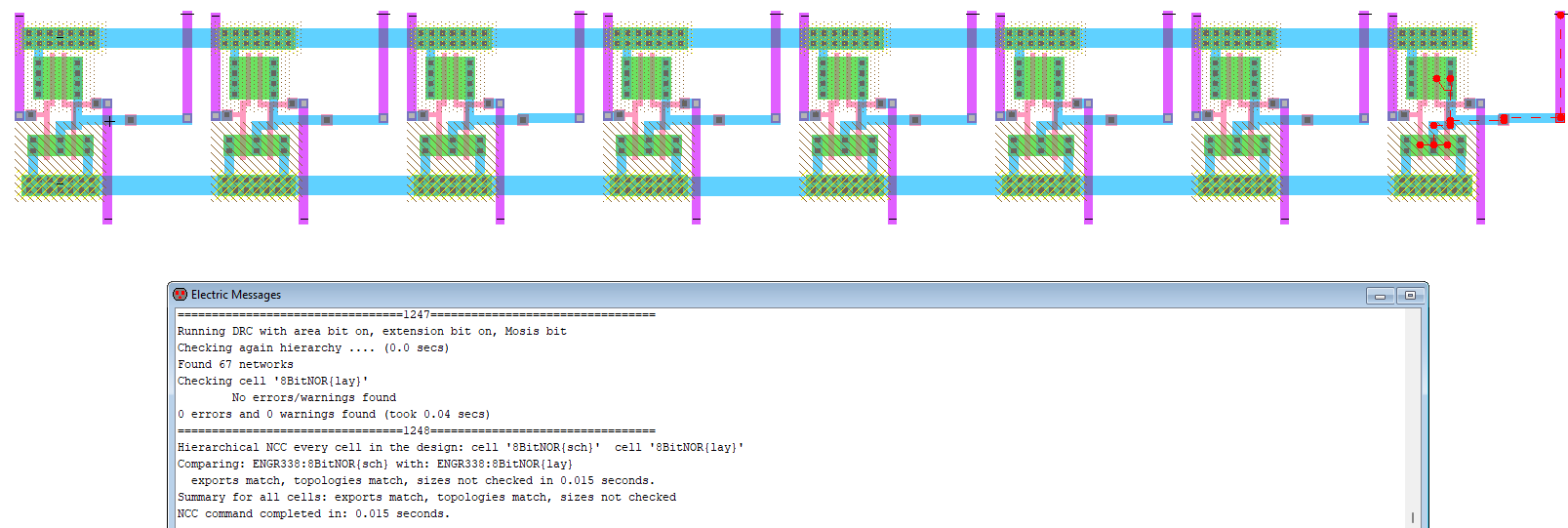Figure 11. 8-bit NAND(top) and 8-bit NOR(bottom) layouts with clean NCC and DRC checks.

With all of the layouts completed, the logic for the gates was tested.

Results:

The NAND, NOR, AND, OR, and Inverter gates were all known to work before this lab so the only thing to test was if the busses were working properly. These tests were performed by shorting the top bus to either ground or VDD and then every other pin to VDD and the others to ground. This test was performed with every gate except for the oscilator.

The ring oscialtor was tested by shorting the ends together and supplying a voltage from 0 to 5V as seen below in Figure 12.Figure 12. Ring oscilator simulation.

The 8-bit AND was then tested with the top pin shorted to VDD and then the top pin shorted to GND. These tests came out with preferable results as seen below, when the top pin was gnd all outputs were near 0V and when it was shorted to VDD the odd numbered pins output VDD as expected.Figure 13. 8-bit AND gate simulation results.

The same process was completed for the 8-bit OR gate where the odd pins should always be at voltage high while the even pins should only be voltage high when the top bus is shorted to VDD.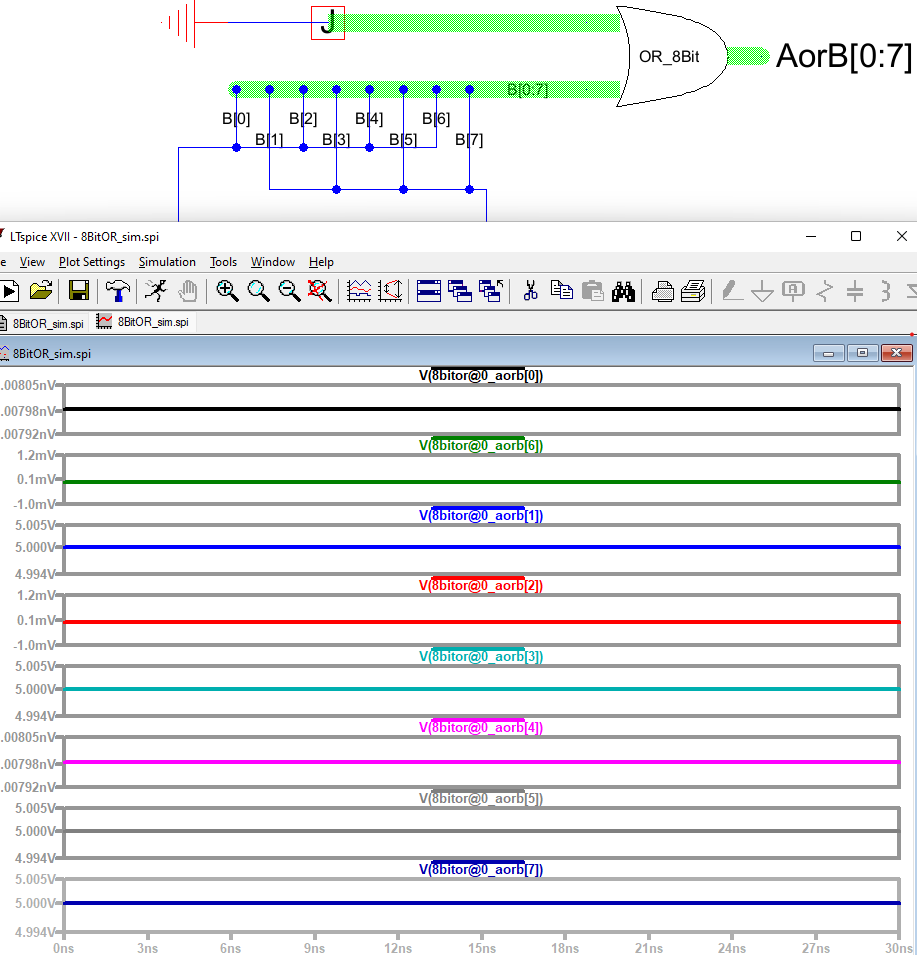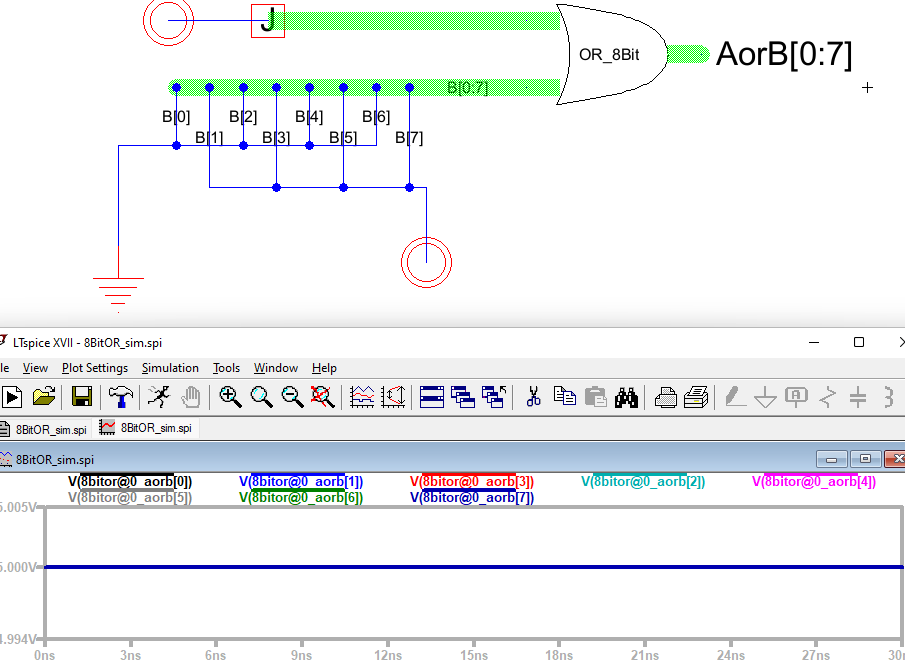Figure 14. 8-bit OR gate simulations.

The same thing was also done for the 8-bit NAND gate where the expected results are that the even pins should always be voltage high(will never be 1 1) and the odd pins should only be 0 when the top bus is shorted to VDD.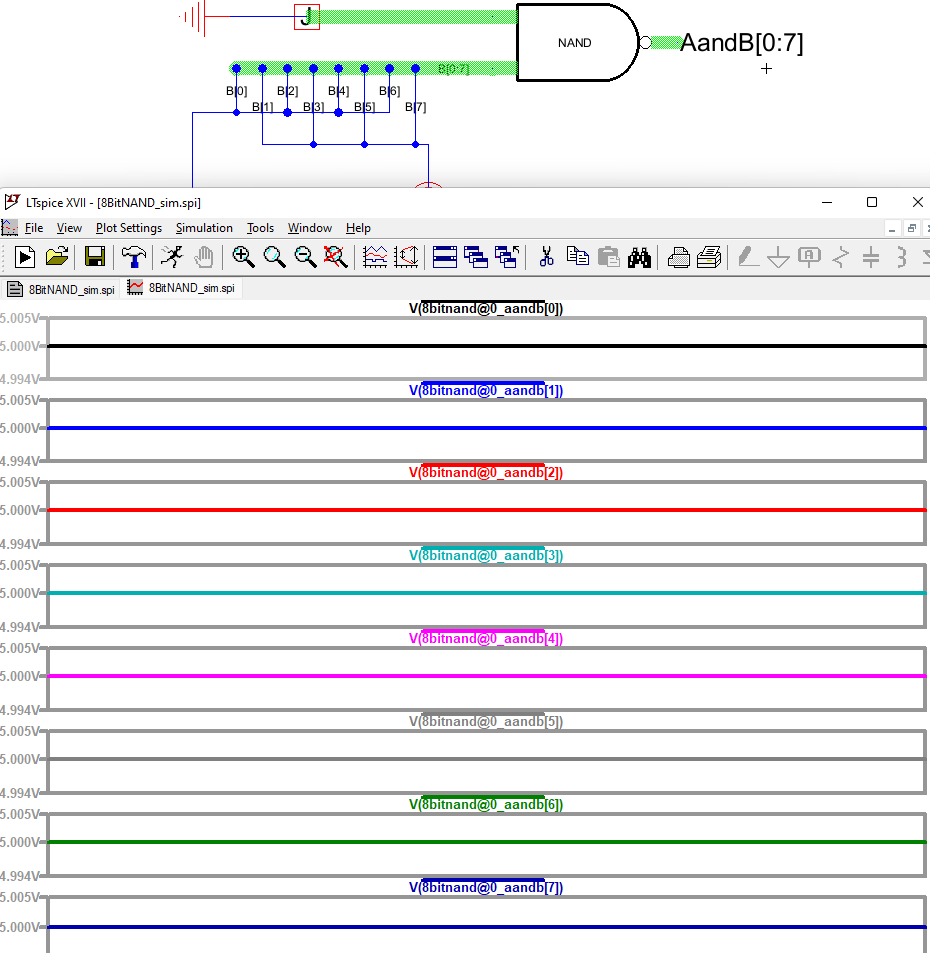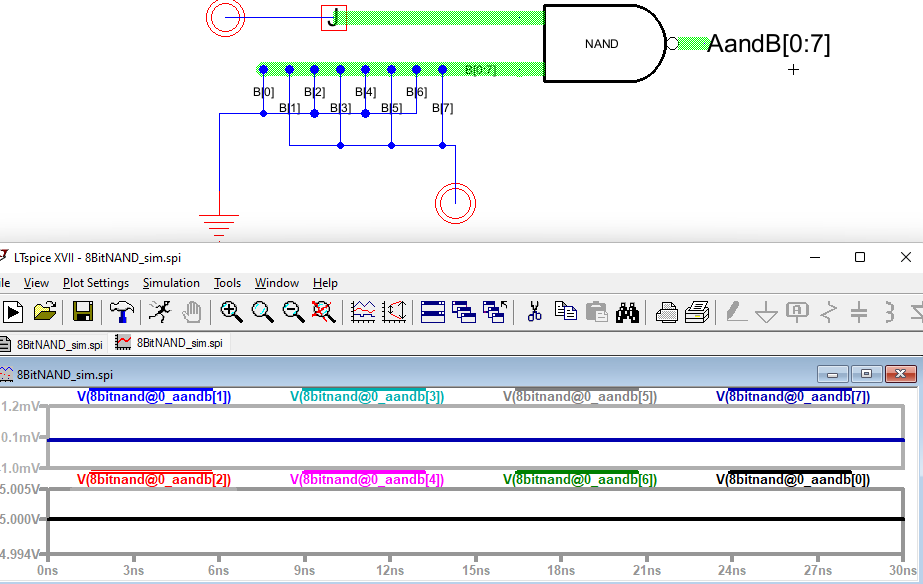Figure 15. 8-bit NAND gate simulation.

Finally, the same thing was done with the NOR gate where the output for the NOR gate should only be voltage high for the even pins when the top pin is shorted to ground and all other cases should be voltage high.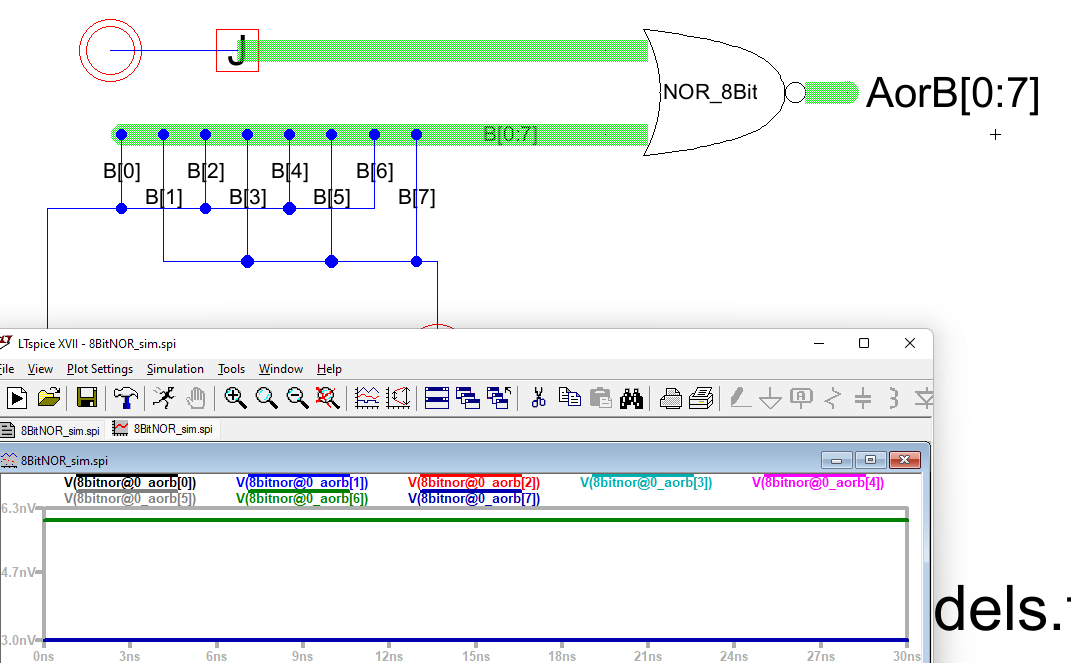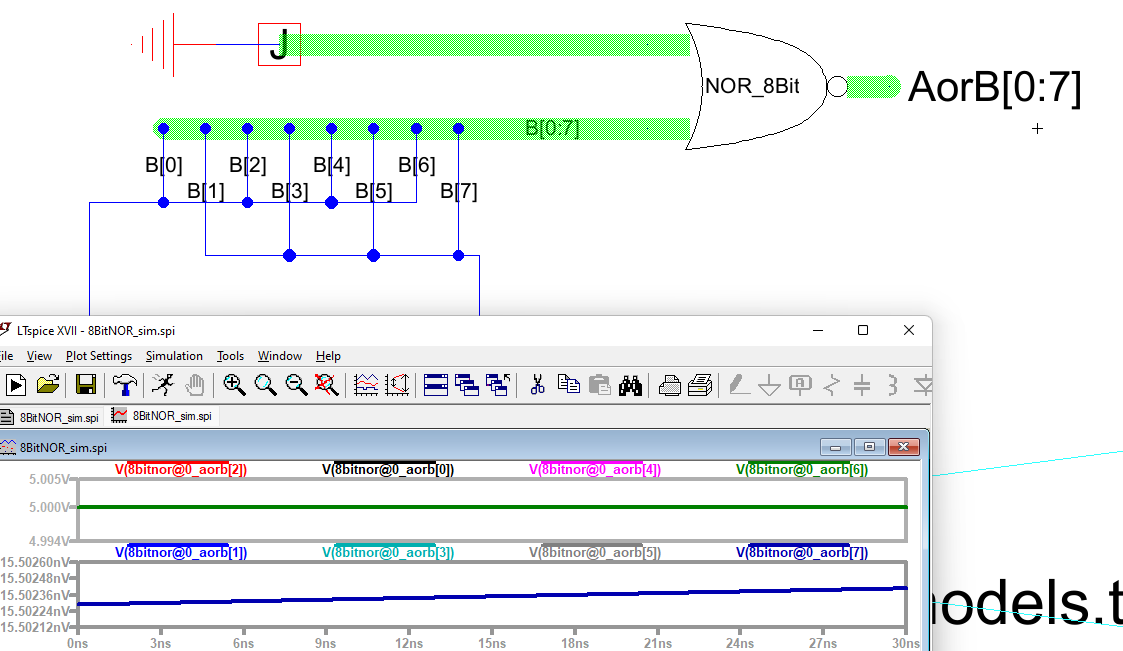Figure 16. 8-bit NOR gate simulation.

Discussion: This lab was ok, it felt repetitive but no part of it was hard just a lot of placing the same wires and running the same tests.